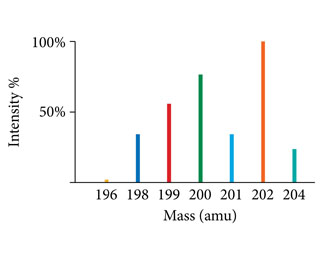# Problem: Use the mass spectrum of mercury to estimate the atomic mass of mercury.Estimate the masses and percent intensity values from the graph to three significant figures.

###### FREE Expert Solution

Mass spectrometry is often used to determine the atomic mass of an element. Recall that the atomic mass of an element depends on the relative abundance of each isotope.

From the figure, we can estimate the intensity of the signal for each isotope:98% (371 ratings)###### Problem Details

Use the mass spectrum of mercury to estimate the atomic mass of mercury.Estimate the masses and percent intensity values from the graph to three significant figures.